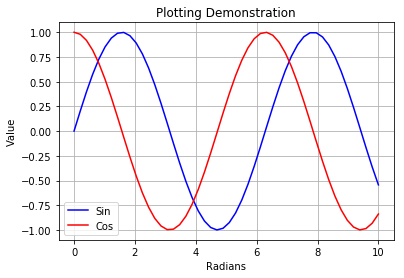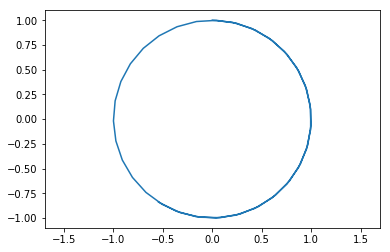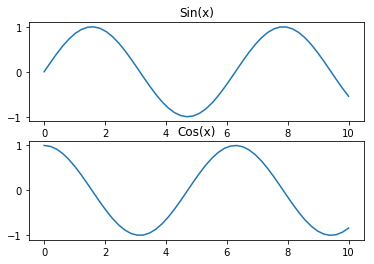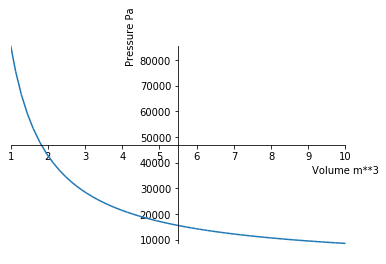This notebook contains course material from CBE20255 by Jeffrey Kantor (jeff at nd.edu); the content is available on Github. The text is released under the CC-BY-NC-ND-4.0 license, and code is released under the MIT license.

# Jupyter Notebooks, Python, and Google Colaboratory¶

## Summary¶

The purpose of this notebook is to introduce Jupyter Notebooks, Python, and Google Colaboratory for routine engineering calculations. This introduction assumes this is your first exposure to these topics.

Jupyter Notebooks are interactive documents, like this one, that include text and code. The documents are ordinary files with a suffix .ipynb. They can be uploaded, downloaded, and shared like any other digital document. The notebooks are composed of individual cells containing either text, code, or the output of a calculation. The code cells be written in different programming languages such as Python, R, and Julia.

Python is a high-level, object-oriented programming language well suited to the rapid development of web and scientific applications. It is widely used for interactive science and engineering computations where productivity is a more important than achieving the highest levels of numerical performance. Python refers to the language itself plus a large eco-system of development tools, documentation, and code libraries for numerical computations, data management, and graphics.

Google Colaboratory is Google's cloud environment for viewing and executing Jupyter/Python notebooks. It operates like Google docs, providing for the collaborative creation and editing of notebooks, and allowing notebooks to be saved to your Google Drive account. Because Colaboratory is based entirely in the cloud, there is no need to install any local software. Colaboratory requires only a browser and internet access to view and execute notebooks.

There other software development environments well suited to viewing and executing the notebooks in this repository. Among them are the excellent distributions

which include tools to view and edit Jupyter notebooks, curated versions of all the major Python code libraries, and additional software development tools. You will want to install one of these distributions if you need off-line access to these notebooks, or if you intend to do more extensive development.

The easiest way to get started with Google Colaboratory is to open a notebook. If you are not currently reading this notebook on Colaboratory, open it here from the github repository.

Google Colaboratory is tightly integrated with Google Drive. Notebooks can be opened directly from Google Drive and automatically saved back to Google Drive. Notebooks can be shared, and support mutiple users collaborating simultaenously the same notebook. These features will be familiar if you've previously used Google Docs. You can obtain a Google Drive or a Google One account here if you don't already have one. The free tier offers 15GB of storage which is enough for routine use of these notebooks.

When opened from a third party resource such as github, the notebook is initially in playground mode. This is fine for viewing notebooks and executing code cells. To save your work you will need to save a copy of the notebook. You can save a copy to:

• a repository in your own github account

## Python Basics¶

Python is an elegant and modern language for programming and problem solving that has found increasing use by engineers and scientists. In the next few cells we'll demonstrate some basic Python functionality.

### Arithmetic Operations¶

Basic arithmetic functions

In :
a = 12
b = 2
print("a + b = ", a + b)
print("a**b = ", a**b)
print("a/b = ", a/b)

a + b =  14
a**b =  144
a/b =  6.0


Most math functions are in the numpy library. This next cell shows how to import numpy with the prefix np, then use it to call a common function

In :
import numpy as np
np.sin(2*np.pi)

Out:
-2.4492935982947064e-16

### Lists¶

Lists are a versatile way of organizing your data in Python. Here are some examples, more can be found on this Khan Academy video.

In :
xList = [1, 2, 3, 4]
print(xList)

[1, 2, 3, 4]


Concatentation is the operation of joining one list to another.

In :
# Concatenation
x = [1, 2, 3, 4];
y = [5, 6, 7, 8];

x + y

Out:
[1, 2, 3, 4, 5, 6, 7, 8]

A common operation is to apply a binary operation to elements of a single list. For an example such as arithmetic addition, this can be done using the sum function from numpy.

In :
# Two ways to sum a list of numbers
print(np.sum(x))

10


A for loop is a means for iterating over the elements of a list. The colon marks the start of code that will be executed for each element of a list. Indenting has meaning in Python. In this case, everything in the indented block will be executed on each iteration of the for loop.

In :
for x in xList:
print("x =", x, "    sin(x) = ", np.sin(x))

x = 1     sin(x) =  0.8414709848078965
x = 2     sin(x) =  0.9092974268256817
x = 3     sin(x) =  0.1411200080598672
x = 4     sin(x) =  -0.7568024953079282


A list comprehension is a very useful tool for creating a new list using data from an existing. For example, suppose you have a list consisting random numbers

In :
from random import random

[i + random() for i in range(0,10)]

Out:
[0.1952325947356377,
1.5664950727528784,
2.8645620994056857,
3.610813783399313,
4.745590841711996,
5.929632460883009,
6.0790789599780926,
7.643940863853748,
8.721811826350383,
9.506420667869708]

### Dictionaries¶

Dictionaries are useful for storing and retrieving data as key-value pairs. For example, here is a short dictionary of molar masses. The keys are molecular formulas, and the values are the corresponding molar masses.

In :
mw = {'CH4': 16.04, 'H2O': 18.02, 'O2':32.00, 'CO2': 44.01}
print(mw)

{'CH4': 16.04, 'H2O': 18.02, 'O2': 32.0, 'CO2': 44.01}


We can a value to an existing dictionary.

In :
mw['C8H18'] = 114.23
print(mw)

{'CH4': 16.04, 'H2O': 18.02, 'O2': 32.0, 'CO2': 44.01, 'C8H18': 114.23}


We can retrieve a value from a dictionary.

In :
mw['CH4']

Out:
16.04

A for loop is a useful means of interating over all key-value pairs of a dictionary.

In :
for species in mw.keys():
print("The molar mass of {:<s} is {:<7.2f}".format(species, mw[species]))

The molar mass of CH4 is 16.04
The molar mass of H2O is 18.02
The molar mass of O2 is 32.00
The molar mass of CO2 is 44.01
The molar mass of C8H18 is 114.23


Dictionaries can be sorted by key or by value

In :
for species in sorted(mw):
print(" {:<8s}  {:>7.2f}".format(species, mw[species]))

 C8H18      114.23
CH4         16.04
CO2         44.01
H2O         18.02
O2          32.00

In :
for species in sorted(mw, key = mw.get):
print(" {:<8s}  {:>7.2f}".format(species, mw[species]))

 CH4         16.04
H2O         18.02
O2          32.00
CO2         44.01
C8H18      114.23


### Plotting¶

Importing the matplotlib.pyplot library gives IPython notebooks plotting functionality very similar to Matlab's. Here are some examples using functions from the

In :
import matplotlib.pyplot as plt
import numpy as np
%matplotlib inline

x = np.linspace(0,10)
y = np.sin(x)
z = np.cos(x)

plt.plot(x,y,'b',x,z,'r')
plt.ylabel('Value');
plt.title('Plotting Demonstration')
plt.legend(['Sin','Cos'])
plt.grid(True)In :
plt.plot(y,z)
plt.axis('equal')

Out:
(-1.09972447591003,
1.0979832896606587,
-1.0992804688576738,
1.0999657366122702)In :
plt.subplot(2,1,1)
plt.plot(x,y)
plt.title('Sin(x)')

plt.subplot(2,1,2)
plt.plot(x,z)
plt.title('Cos(x)')

Out:
Text(0.5, 1.0, 'Cos(x)')## Solving Equations using Sympy¶

One of the best features of Python is the ability to extend it's functionality by importing special purpose libraries of functions. Here we demonstrate the use of a symbolic algebra package Sympy for routine problem solving.

In :
import sympy as sym

sym.var('P V n R T');

# Gas constant
R = 8.314        # J/K/gmol
R = R * 1000     # J/K/kgmol

# Moles of air
mAir = 1         # kg
mwAir = 28.97    # kg/kg-mol
n = mAir/mwAir   # kg-mol

# Temperature
T = 298

# Equation
eqn = sym.Eq(P*V,n*R*T)

# Solve for P
f = sym.solve(eqn,P)
print(f)

# Use the sympy plot function to plot
sym.plot(f,(V,1,10),xlabel='Volume m**3',ylabel='Pressure Pa')

85521.9882637211/VOut:
<sympy.plotting.plot.Plot at 0x12027dac8>

Many of the physical properties of chemical species are given as functions of temperature or pressure.

Python offers a full range of programming language features, and there is a seemingly endless range of packages for scientific and engineering computations. Here are some suggestions on places you can go for more information on programming for engineering applications in Python.

### Tutorial Introductions to Python for Science and Engineering¶

This excellent introduction to python is aimed at undergraduates in science with no programming experience. It is free and available at the following link.

The following text is licensed by the Hesburgh Library for use by Notre Dame students and faculty only. Please refer to the library's acceptable use policy. Others can find it at Springer or Amazon. Resources for this book are available on github.

pycse is a package of python functions, examples, and document prepared by John Kitchin at Carnegie Mellon University. It is a recommended for its coverage of topics relevant to chemical engineers, including a chapter on typical chemical engineering computations.